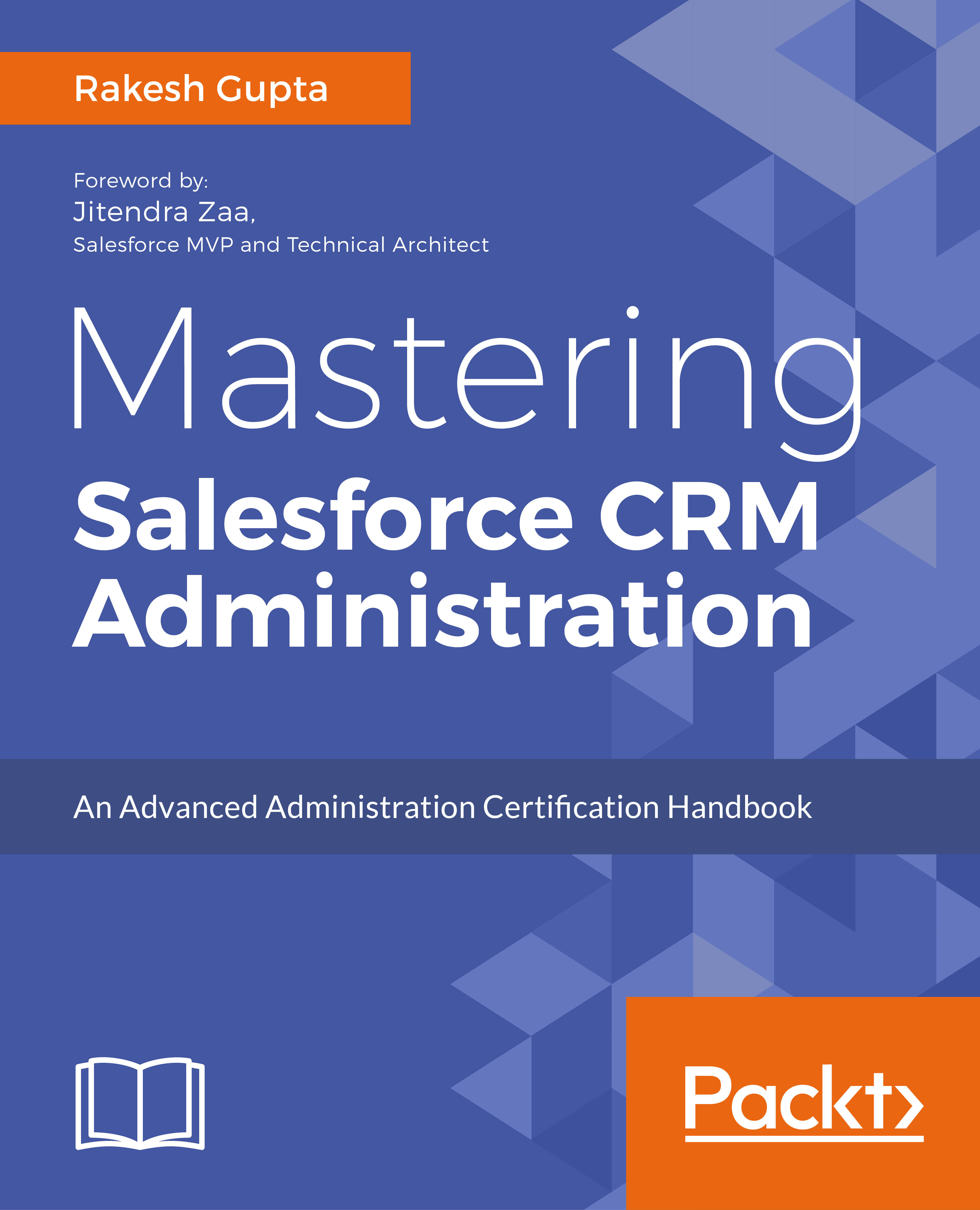• ## Quick-guide to VLOOKUP for automation experts

##### Posted at 7:29 PM by Rakesh Gupta, on June 5, 2017

*This post was contributed by guest author Hannah Sharron

VLOOKUP is one of the most well-known Excel functions – and not without reason!If I had to tell the most used function in excel, I would say VLOOKUPMany people would even say, that you have not learned Excel if you can’t perform a simple (V)LOOKUP. So, let us see what VLOOKUP is all about.

VLOOKUP has a super high versatile usage. This is a great thing, but many people find it hard to implement the function in practice.

VLOOKUP’s ease of use and simplicity when looking up data is unparalleled in Excel.

• Maybe you want to learn the basics of VLOOKUP step-by-step
• Perhaps your VLOOKUP formula isn’t working?

If yes, then you’ve come to the right place! Therefore, I’m going to give you a quick rundown on how to use VLOOKUP in a simple way.

Let’s look at an example:

How would you fill in data in the green cell in Table 2 using data from Table 1?## The Logic

Well, one simple approach is to use VLOOKUP. To do that let us first understand this function.

VLOOKUP or ‘Vertical Lookup’ helps in ‘looking up in vertical direction’ (hence the name VLOOKUP) a value in a data array or data organized in rows and columns.

Once it locates the ‘look-up-value’, corresponding column values can be pulled out.

So, if we wish to fill the green cells in Table 2 with a name (say Lee Nazal), we start by asking VLOOKUP to look for the name ‘Lee Nazal’ in Table 1 (which is row 9). Then VLOOKUP is to give us the corresponding value in the columns “Department” (which is the second column in Table 1) and “Salary” (which is column 3).

This is essentially how the logic of VLOOKUP works.

## Formula

Now we know the logic, here is how the formula looks like (also called its syntax):VLOOKUP is made up of 4 important fields, namely:

1. lookup_value: The value you want to look up in a data array and find its corresponding column values (i.e. cell E3). Read the rest of this entry!

• #### Sponsor #1• #### Sponsor #2• #### Sponsor #3• #### Sponsor #4• #### Sponsor #5• #### Buy My Book• #### Buy My Book• #### Buy My Book